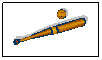# Mr. Penza, Instructor

A Technology Plan with elements of Mathematical Thinking,

Microsoft Excel, and Baseball Stat Calculation.

Designed for a Middle to High School classroom.

The NCTM Framework Standards employed:

·        Develop fluency in operations with real numbers, vectors, and matrices, using mental computation or paper-and-pencil calculations for simple cases and technology for more-complicated cases.

·        Judge the reasonableness of numerical computations and their results

·        Draw reasonable conclusions about a situation being modeled

·        Compute basic statistics and understand the distinction between a statistic and a parameter.

Lesson Objective:  To introduce the student to the relationship of MLB batting statistics, and then incorporate this understanding by applying formula concepts and analysis in a Microsoft Excel Spreadsheet.2.  Now click on this link.   This is the Los Angeles Dodgers web site.   Search around to find the team statistics page.   You will need this page to gather input for your calculations.   Print out the page so you can refer back.

3. Now click the Calculator on the Navigator bar (left).   Try out some numbers to see how it works.

4. See if you can figure out how the Calculator is figuring each of the OUTPUT values.

5. Are some of your calculated values not exactly correct?  Why do you think that is?

6.  Once you are comfortable with the statistics, click on the Submit button (left),  answer the questions and submit to your instructor.

20 POSSIBLE POINTS

 SCORE UNDERSTANDING EXCEL FORMULAS WORKSHEET Q & A COMMENTS 4 Student can execute and explain all formulas with high efficiency. Outstanding effort on task. Quickly figures formulas with accuracy. Consistent Performance Excellent answers provided. 3 Student can develop formula but has a little trouble communicating the concepts. Formulas are slowly understood with extensive concentration. Good answers. Correct most of the time.  Some error in logic. 2 Student has trouble completing all formulas, but seems to communicate a general understanding. Formulas are not completely understood.  Effort is being made. Averages in the 50% range for accuracy.  More effort required to grasp concepts. 1 Student is not able to develop the formula to arrive at an answer. No sign of formula knowledge or effort. No valid answers supplied.  Poor effort. TOTALS